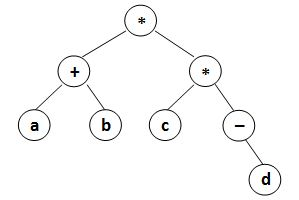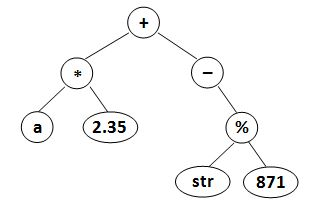Given a syntax tree (binary), you are supposed to output the corresponding postfix expression, with parentheses reflecting the precedences of the operators.

Input Specification:
Each input file contains one test case. For each case, the first line gives a positive integer N (≤ 20) which is the total number of nodes in the syntax tree. Then N lines follow, each gives the information of a node (the i-th line corresponds to the i-th node) in the format:

data left_child right_child
where data is a string of no more than 10 characters, left_child and right_child are the indices of this node's left and right children, respectively. The nodes are indexed from 1 to N. The NULL link is represented by −1. The figures 1 and 2 correspond to the samples 1 and 2, respectively.Figure 1Figure 2
Output Specification:
For each case, print in a line the postfix expression, with parentheses reflecting the precedences of the operators.There must be no space between any symbols.

Sample Input 1:
8
* 8 7
a -1 -1
* 4 1
+ 2 5
b -1 -1
d -1 -1
- -1 6
c -1 -1

## Sample Output 1:

(((a)(b)+)((c)(-(d))))

8
2.35 -1 -1
* 6 1
- -1 4
% 7 8
+ 2 3
a -1 -1
str -1 -1
871 -1 -1

## Sample Output 2:

(((a)(2.35)*)(-((str)(871)%))+)

## 解答

#include<iostream>
#include<vector>
#include<string>

using namespace std;

struct node {
string data;
int lchild, rchild;
};

vector<node> nodes;

string postOrder(int root) {
if (root == -1) return "";
int lchild = nodes[root].lchild, rchild = nodes[root].rchild;
//if (lchild == -1 && rchild == -1)
//	return nodes[root].data;
if (lchild != -1 && rchild != -1)
return "(" + postOrder(lchild) + postOrder(rchild) + nodes[root].data + ")";
else //if (rchild != -1)
return "(" + nodes[root].data + postOrder(rchild) + ")";
//else
//	return "(" + postOrder(lchild) + nodes[root].data + ")";
}

int main() {
int n, root;
scanf("%d", &n);
vector<bool> isRoot(n + 1, true);
nodes.resize(n + 1);
for (int i = 1; i <= n; i++) {
cin >> nodes[i].data >> nodes[i].lchild >> nodes[i].rchild;
//if (nodes[i].data != "+" && nodes[i].data != "-" &&nodes[i].data != "%" && nodes[i].data != "*")
//	nodes[i].data = "(" + nodes[i].data + ")";
if (nodes[i].lchild != -1) isRoot[nodes[i].lchild] = false;
if (nodes[i].rchild != -1) isRoot[nodes[i].rchild] = false;
}
for (int i = 1; i <= n; i++) {
if (isRoot[i]) {
root = i;
break;
}
}
printf("%s", postOrder(root).c_str());
return 0;
}